Function Repository Resource:

# BoundaryElementMeshJoin

Join multiple boundaries to form a single boundary ElementMesh

Contributed by: Oliver Rübenkönig
 ResourceFunction["BoundaryElementMeshJoin"][bmesh1,bmesh2,…] returns a boundary ElementMesh consisting of joined boundary MeshRegion or ElementMesh objects bmeshi.

## Details and Options

ResourceFunction["BoundaryElementMeshJoin"] accepts the options for ToBoundaryMesh.
Inputs can be either MeshRegion or ElementMesh objects.

## Examples

### Basic Examples (4)

Load the Finite Element Method package:

 In:=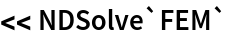Define two boundary meshes:

 In:=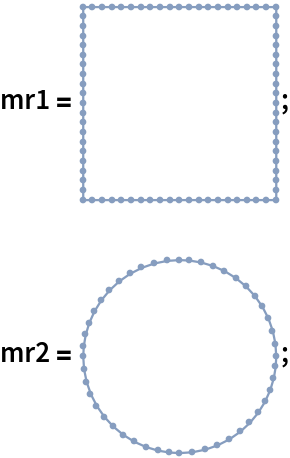Join the two:

 In:=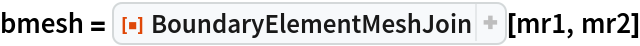Out=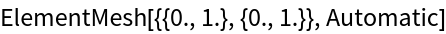Visualize the resulting boundary ElementMesh:

 In:=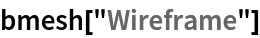Out=### Scope (7)

Create boundary element meshes directly:

 In:=Join the boundary meshes:

 In:=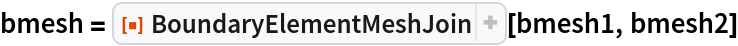Out=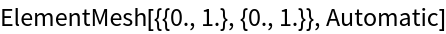Visualize the resulting boundary ElementMesh:

 In:=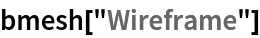Out=Generate a full ElementMesh with the internal circle boundary:

 In:=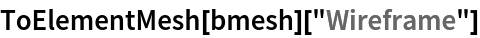Out=Create three boundary meshes:

 In:=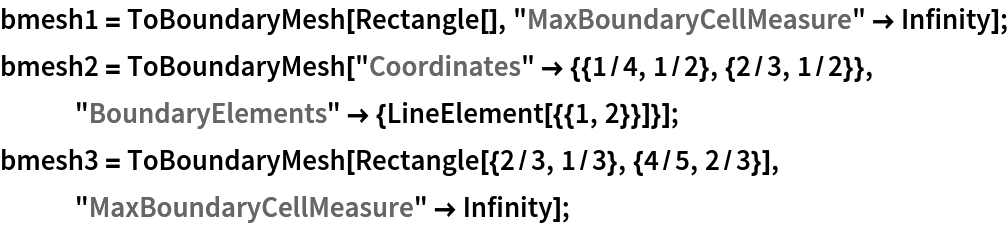Join the boundary meshes:

 In:=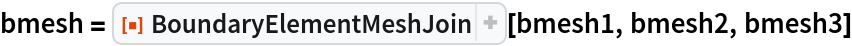Out=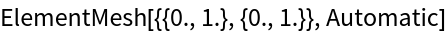Visualize the resulting boundary ElementMesh:

 In:=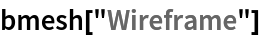Out=Generate a full ElementMesh with the internal line boundary and a region hole in the box:

 In:=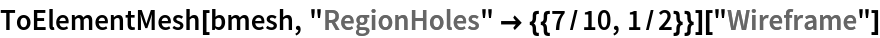Out=Create two boundary meshes:

 In:=Join the boundary meshes:

 In:=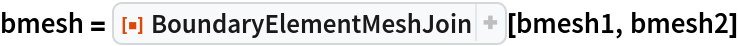Out=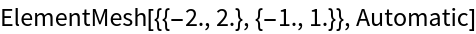Visualize the resulting boundary ElementMesh:

 In:=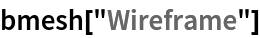Out=Generate a full ElementMesh with the internal boundaries:

 In:=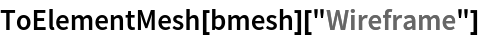Out=Create two boundary meshes:

 In:=Join the boundary meshes:

 In:=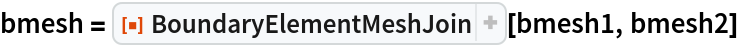Out=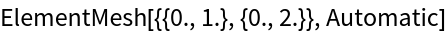Visualize the resulting boundary ElementMesh:

 In:=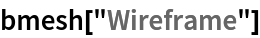Out=Generate a full ElementMesh with an internal boundary at the connection:

 In:=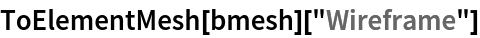Out=Create two boundary meshes:

 In:=Join the boundary meshes:

 In:=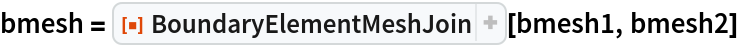Out=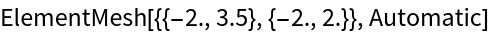Visualize the resulting boundary ElementMesh:

 In:=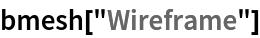Out=Generate a full ElementMesh with the internal boundaries:

 In:=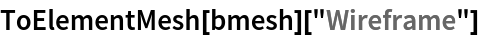Out=Generate a full ElementMesh with the internal boundaries and a region hole:

 In:=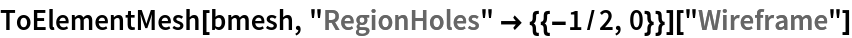Out=Create two boundary meshes in 3D:

 In:=Join the boundary meshes:

 In:=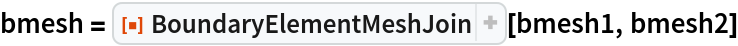Out=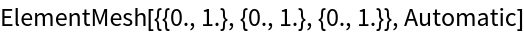Visualize the resulting boundary ElementMesh:

 In:=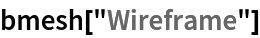Out=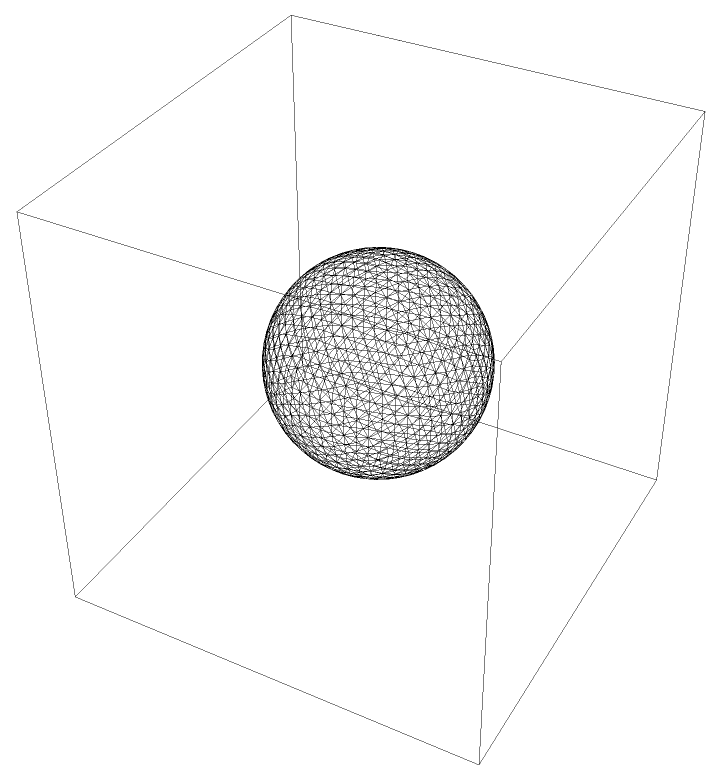Generate a full ElementMesh with the internal ball boundary:

 In:=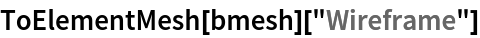Out=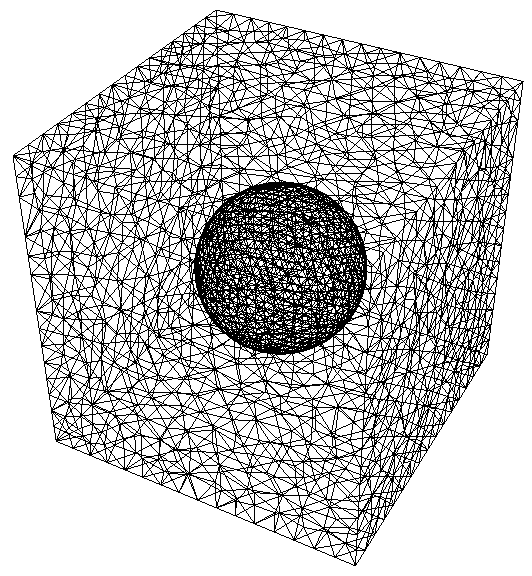Create two boundary meshes in 3D:

 In:=Join the boundary meshes:

 In:=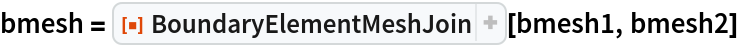Out=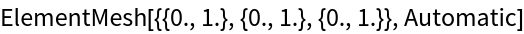Visualize the resulting boundary ElementMesh:

 In:=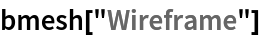Out=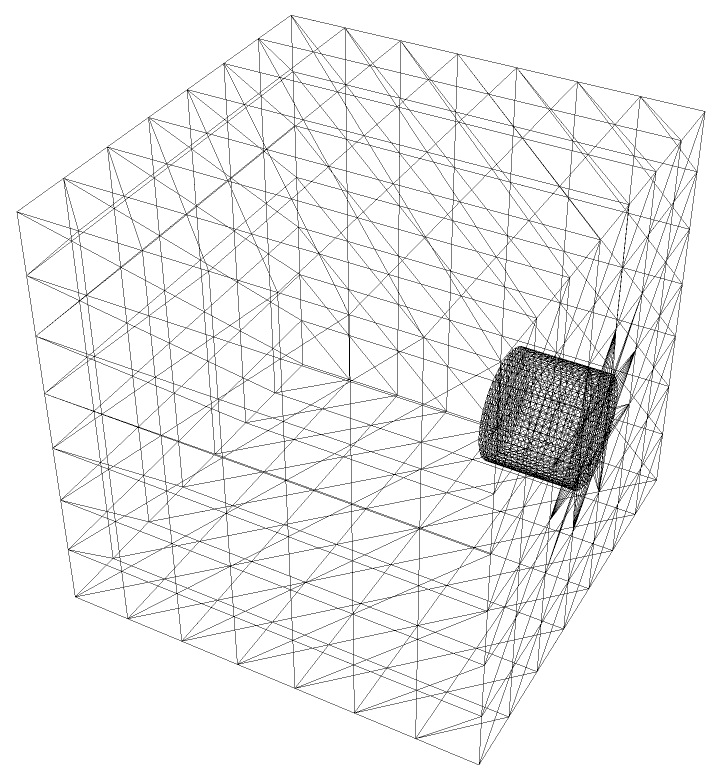Generate a full ElementMesh:

 In:=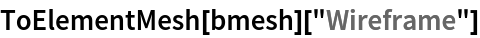Out=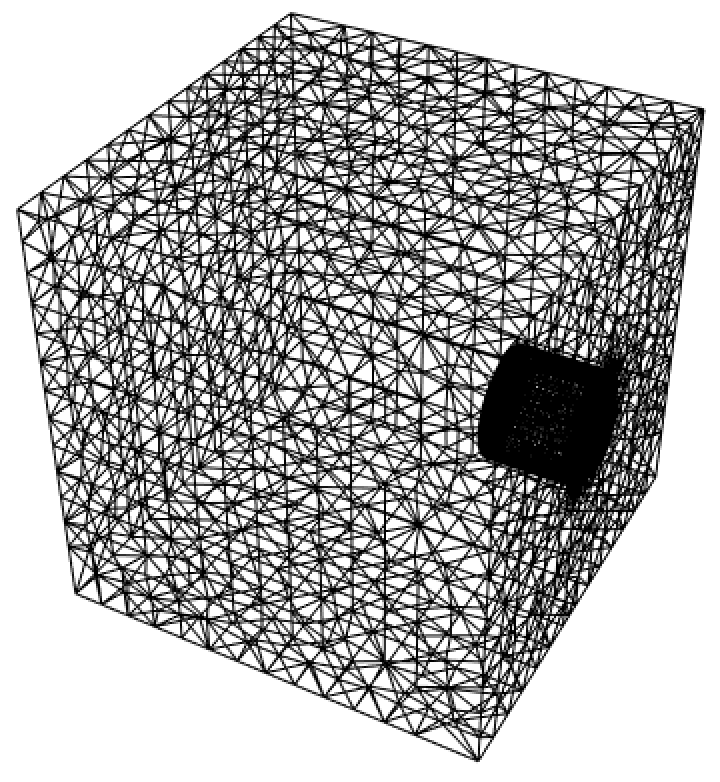### Applications (2)

Use an image to generate a mesh that has material regions. Set up the image:

 In:=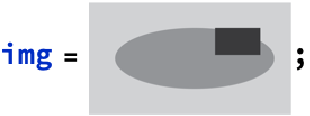Find the image components:

 In:=Generate mesh regions:

 In:=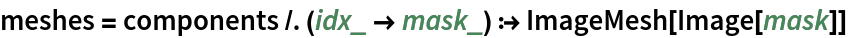Out=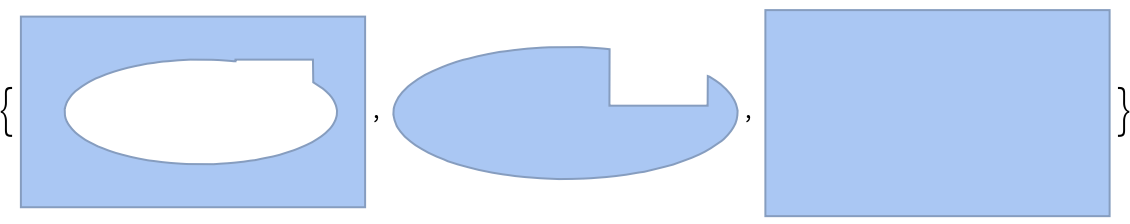Generate boundary ElementMesh objects and join them:

 In:=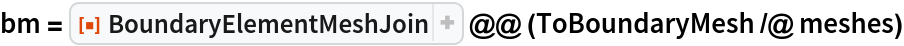Out=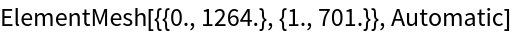Visualize the boundary ElementMesh:

 In:=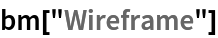Out=Find the centroids of the meshes:

 In:=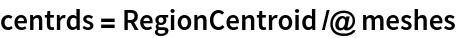Out=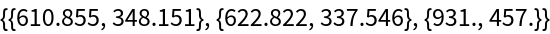Generate the full ElementMesh with region markers:

 In:=Out=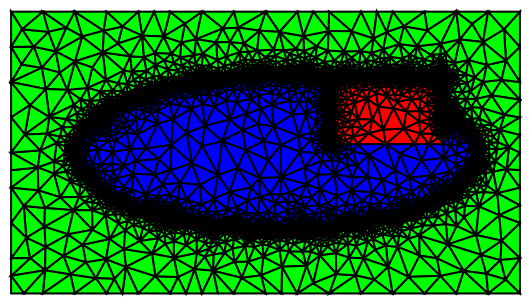Solve a PDE with different coefficient values in the different material regions:

 In:=Visualize the solution:

 In:=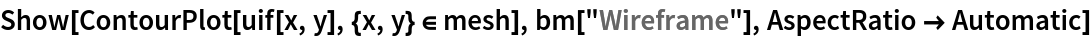Out=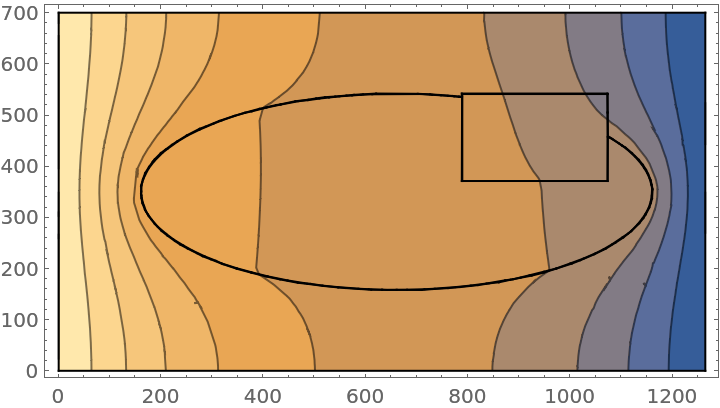Create two boundary meshes in 3D:

 In:=Join the meshes:

 In:=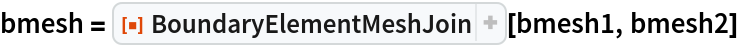Out=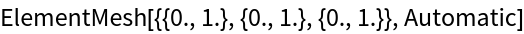Visualize the boundary ElementMesh:

 In:=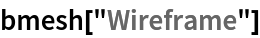Out=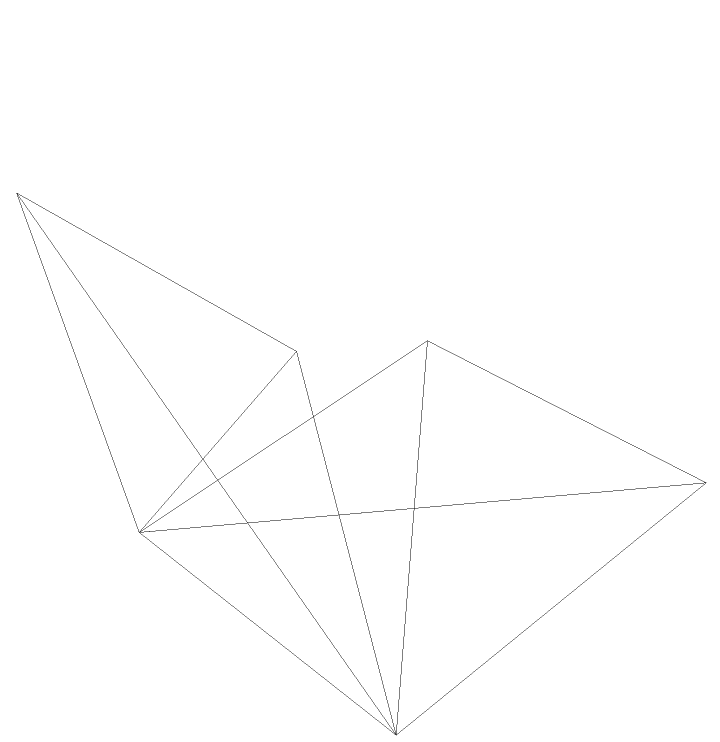Numerically integrate over the boundary ElementMesh:

 In:=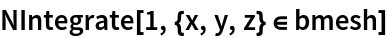Out=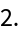### Possible Issues (6)

Sometimes the boundary mesh quality is not good enough to generate a full ElementMesh. Create two boundary ElementMesh objects:

 In:=Join the boundary meshes:

 In:=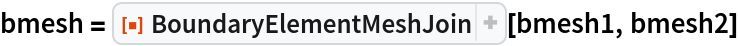Out=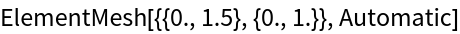Visualize the resulting boundary ElementMesh:

 In:=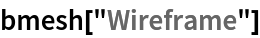Out=Generate a full ElementMesh with the internal circle boundary:

 In:=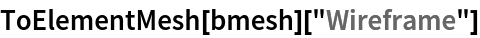Out=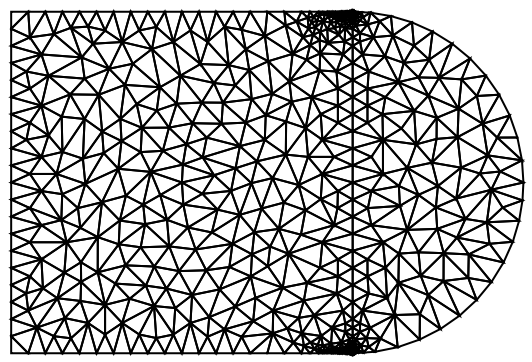In that case, generate a small offset at the intersecting boundary:

 In:=Generate a full ElementMesh with the internal circle boundary:

 In:=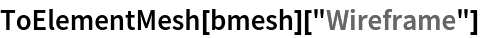Out=OliverRubenkonig

## Version History

• 1.0.0 – 20 January 2020